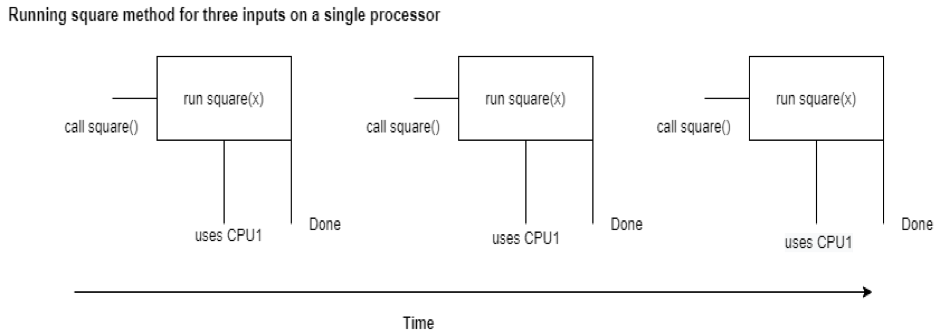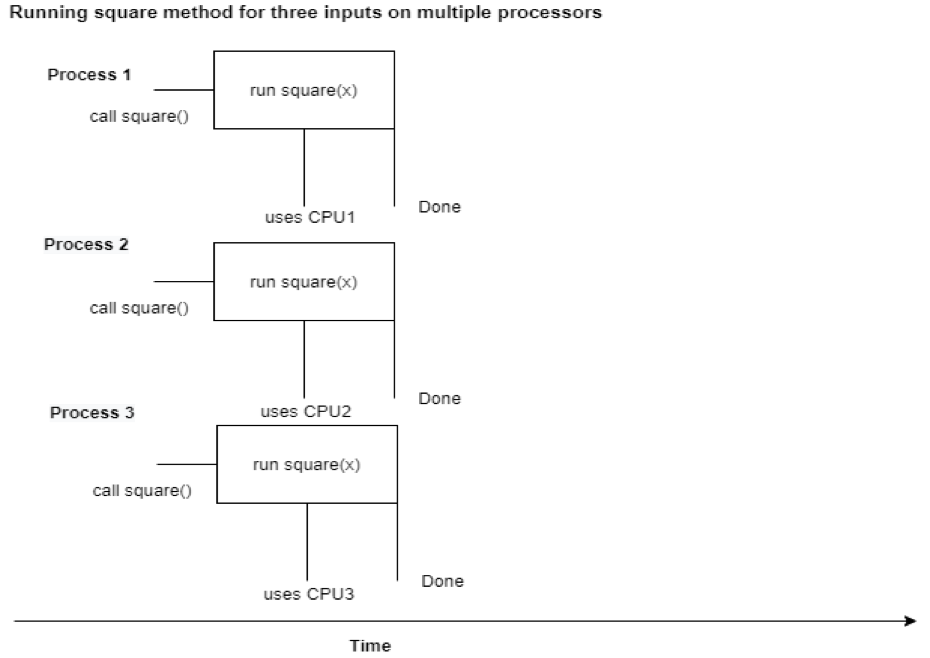# Introduction to Multiprocessing and Process in Python

June 23, 2020 8189

Today, computers no longer have only one CPU core or processor. They usually have more than one central processing unit or more than one core in a CPU. According to Wikipedia, “Multiprocessing is the use of two or more central processing units (CPUs) within a single computer system”. Multiprocessing can significantly increase the performance of the program. Let’s consider an example to understand this topic. Suppose you want to find out the numbers that are divisible by 3, between 1 and 100. Now, you are the only person to do this task. You will have to, individually, check for each number one by one. Consider another scenario in which your friend wants to help you out. You check the first 50 numbers, while your friend checks the other half, simultaneously. The amount of time, in this scenario, is reduced by half. A similar procedure happens in multiprocessing. i.e. instead of one processor doing the whole task, multiprocessors do the parts of a task simultaneously.

Unlike C or Java that makes use of multiprocessing automatically, Python only uses a single CPU because of GIL (Global Interpreter Lock). It is a lock that only allows one thread to execute at one time. However, Python’s multiprocessing module can deal with that problem. This module contains two classes, the Process and the Pool that can allow us to run a certain section of code simultaneously. In this tutorial, we are going to look at the Process class in detail.

First, we will take an example that solves a problem serially. Then, we will use multiprocessing to parallelize it.import time

def square(x):
print(f"start process:{x}")
square = x * x
print(f"square {x}:{square}")
time.sleep(1)
print(f"end process{x}")

starttime = time.time()
for i in range(0, 5):
square(i)

endtime = time.time()
print(f"Time taken {endtime-starttime} seconds")


Output

start process:0 square 0:0 end process:0 start process:1 square 1:1 end process:1 start process:2 square 2:4 end process:2 start process:3 square 3:9 end process:3 start process:4 square 4:16 end process:4 Time taken 5.008170127868652 seconds

We have a function square(x) that takes the square of a number and sleeps for 1 second. This function runs 5 times and takes the square of the number from 0 to 5(exclusive). All this work is done serially. In the output, you can see that only when the function is done running for input 0, it starts running for input 1, and so on. The amount of time taken to perform the whole task is approximately 5 seconds.

Let’s see how we can speed this up using the Process class of the multiprocessing module.

import time
from multiprocessing import Process

def square(x):
print(f"start process:{x}")
square = x * x
print(f"square {x}:{square}")
time.sleep(1)
print(f"end process:{x}")

if __name__ == "__main__":
starttime = time.time()
processlist = []
for i in range(0, 5):
process = Process(target=square, args=(i,))
processlist.append(process)
process.start()

for process in processlist:
process.join()
endtime = time.time()
print(f"Time taken {endtime-starttime} seconds")


Output

start process:0 square 0:0 start process:1 start process:2 start process:3 square 2:4 square 3:9 square 1:1 start process:4 square 4:16 end process:0 end process:3 end process:2 end process:1 end process:4 Time taken 1.0663228034973145 seconds

Let’s go through this line by line to understand what is happening.

In the first line, we import the time module to calculate the running time and to sleep for 1 second.

In the second line, we import Process class from the multiprocessing module to create processes and parallelize the program.

Next, we have the square() function, which performs the same task as it did in the previous example. In the main part, we start the time and create a list where we will store the processes.

In the for loop, we create five processes, append each process to processlist and start the processes.

To create a process, we call the constructor of the Process class where we provide two arguments. i.e. Process(target=square, args=(i,)). The target argument represents the section of code to be executed by the created process and the args argument is used to pass the argument(s) to the function if any. Process.start() is used to start a process.

The main python script is a Parent process, and the processes created are the child processes that can inherit necessary resources to execute the task, inherit all the resources, etc. depending upon which start method your platform is using. Check Start Methods.

The process.join() method blocks the parent process and waits for the process to finish its execution. It also takes an optional timeout argument (default value is None), which will wait for maximum timeout seconds for the process to finish. It returns if the process terminates or the timeout limit reaches. A process cannot join itself as it will cause a deadlock. i.e. A process has been blocked and waiting for itself to finish its execution.

In the end, we calculate the end time and print the total execution time. In the output, we can see that it only takes approximately 1 second, which is 5 times less than the previous example.

As we know that the parent process and the child process are two different processes. Therefore, they have different process identification numbers or pids. Let’s see.

import time
from multiprocessing import Process
import os

def test():
print("start test process")
time.sleep(1)
print(f"Id of the child process in the test function {os.getpid()}")
print("end test process")

if __name__ == "__main__":
print(f"Id of the parent process {os.getpid()}")
process = Process(target=test)
process.start()

print(f"Id of the child process {process.pid}")
process.join()


Output

Id of the parent process 123 start test process Id of the child process 322 Id of the child process in the test function 322 end test process

We can see that the pid of the parent process is different from the child process. os.getpid() returns the ID of the current process and process.pid returns the ID of the process.

The process.is_alive() method checks if the process is alive or not, i.e., if it is currently in execution. It returns True if it is in execution. Otherwise, False.

import time
from multiprocessing import Process
import os

def test():
print("start test process")
time.sleep(1)
print("end test process")

if __name__ == "__main__":
process = Process(target=test)
print(f"The process is alive:{process.is_alive()}")
process.start()
print(f"The process is alive:{process.is_alive()}")
process.join()
print(f"The process is alive:{process.is_alive()}")


Output

The process is alive:False start test process The process is alive:True end test process The process is alive:False The process is alive after it has started and before it has terminated.

What if the child process wants to send some data to the parent process, let’s say, the square of the numbers? Parent and child are two different processes, and hence, they have different memory segments. Therefore, we need some kind of communication channel to pass data from one process to another. multiprocessing supports two types of communication channel between processes.

## Queue

A Queue can be used to pass messages between processes. It is a near clone of queue.Queue. The syntax is multiprocessing.Queue(maxsize). The argument maxsize is optional. Let’s see an example to understand this.

import time
from multiprocessing import Process, Queue

def square(li, q):
for x in li:
square = x * x
q.put(square)

if __name__ == "__main__":
li = range(0, 5)
q = Queue()
process = Process(target=square, args=(li, q))
process.start()
process.join()
while not q.empty():
print(q.get())


Output

0 1 4 9 16

We put the square of each number from 1 to 5(exclusive) in the queue using the q.put() method. After the child process has finished its execution, we get the data from the queue until it is empty using the q.get() method.

## Pipe

While the Queue can have multiple producers and consumers, Pipe can only have two endpoints. The syntax is multiprocessing.Pipe(duplex). The optional duplex argument shows that the pipe is bidirectional or unidirectional. By default, it is True(bidirectional). This method returns a pair of connection objects for each endpoint i.e. (connection1, connection2). If the duplex argument is set to False, then connection1 can only receive messages, and connection2 can only send messages. Let’s do the same example using a pipe.

import time
from multiprocessing import Process, Pipe

def square(li, con2):
for x in li:
square = x * x
con2.send(square)

if __name__ == "__main__":
li = range(0, 5)
con1, con2 = Pipe()
process = Process(target=square, args=(li, con2))
process.start()

for i in range(0, 5):
print(con1.recv())
process.join()


Output

0 1 4 9 16

The child process is sending data using con2.send(), and the parent process is receiving data using con1.recv(). Note that if both processes send or receive data from the same end of the pipe at the same time, the data in a pipe can be corrupted

Liked the post?
A computer science student having interest in web development. Well versed in Object Oriented Concepts, and its implementation in various projects. Strong grasp of various data structures and algorithms. Excellent problem solving skills.
0 COMMENT